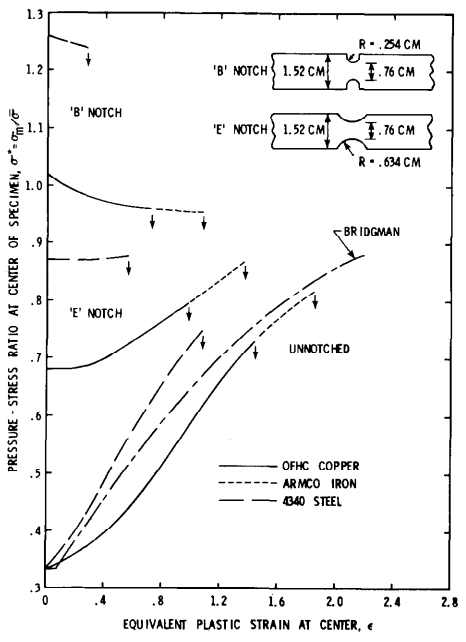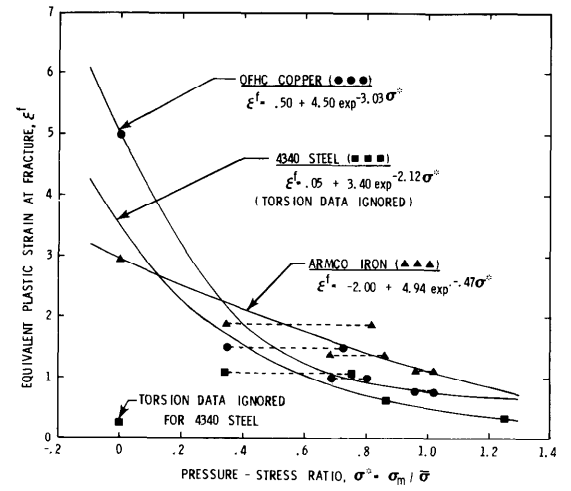# JC失效模型参数的确定

$$\sigma _{Y}= \left (A+B\bar{\varepsilon _{p}}^{n} \right )\left ( 1+Cln\dot{\varepsilon }^{*} \right )\left ( 1-T^{*} \right )$$

$$\varepsilon ^{f}= \left [ D_{1}+D_{2}e^{D_{3}\sigma ^{*}} \right ]\left [ 1+D_{4}ln\dot{\varepsilon } ^{*}\right ]\left [ 1+D_{5}T^{*} \right ]$$

$$D=\sum \frac{\Delta \varepsilon _{p}}{\varepsilon ^{f}}$$

#### 3.拟合D1、D2和D3#### 4.拟合D4和D5Johnson G R, Cook W H. Fracture characteristics of three metals subjected to various strains, strain rates, temperatures and pressures[J]. Engineering fracture mechanics, 1985, 21(1): 31-48.